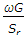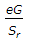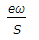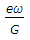# Civil Engineering - Soil Mechanics and Foundation Engineering

### Exercise :: Soil Mechanics and Foundation Engineering - Section 2

16.

The earth pressure of a soil at rest, is proportional to (where φ is the angle of internal friction of the soil)

 A. tan (45° - φ) B. tan (45° + φ) C. tan2 (45° - φ) D. tan2 (45° + φ) E. none of these

Explanation:

No answer description available for this question. Let us discuss.

17.

A fundamental equation of void ratio(e), specific gravity (G), water content (ω) and degree of saturation (Sr) is

 A. e =B. ω =C. G =D. Sr =Explanation:

No answer description available for this question. Let us discuss.

18.

The critical exist gradient of seepage water in soils, is

 A. directly proportional to the voids ratio B. inversely proportional to the specific gravity C. directly proportional to the specific gravity D. inversely proportional to the voids ratio E. none of these.

Explanation:

No answer description available for this question. Let us discuss.

19.

Water content of a soil sample is the difference of the weight of the given sample at the given temperature and the weight determined after drying it for 24 hours at temperature ranging from

 A. 80° to 90°C B. 90° to 95°C C. 95° to 100°C D. 103° to 105°C E. 105° to 110°C

Explanation:

No answer description available for this question. Let us discuss.

20.

The ratio of the volume of voids to the total volume of the given soil mass, is known

 A. porosity B. specific gravity C. void ratio D. water content.